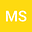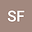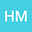A new treatment of convex functions
•••• Shigeru Furuichi,
Princess Sumaya University for Technology

Corresponding Author:[email protected]

Author ProfileShigeru Furuichi
Nihon Sekijujisha
Author ProfileConvex functions have played a major role in the field of Mathematical inequalities. In this paper, we introduce a new concept related to convexity, which proves better estimates when the function is somehow more convex than another.\\ In particular, we define what we called $g-$convexity as a generalization of $\log-$convexity. Then we prove that $g-$convex functions have better estimates in certain known inequalities like the Hermite-Hadard inequality, super additivity of convex functions, the Majorization inequality and some means inequalities.\\ Strongly related to this, we define the index of convexity as a measure of “how much the function is convex”.\\ Applications including Hilbert space operators, matrices and entropies will be presented in the end.